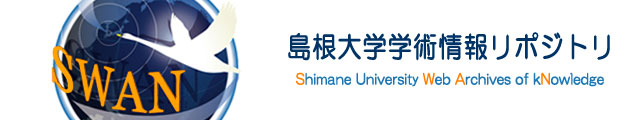language eng Author Ishibashi, Kazuki Department of Electronic Control Engineering, National institute of Technology, Hiroshima College, Description As is well known, Mathieu’s equation is a representative of mathematical models describingparametric excitation phenomena. This paper deals with the oscillation problem forMathieu’s equation with two frequencies. The ratio of these two frequencies is not necessarilya rational number. When the ratio is an irrational number, the coefficient of Mathieu’sequation is is quasi-periodic, but not periodic. For this reason, the basic knowledgefor linear periodic systems such as Floquet theory is not useful. Whether all solutions ofMathieu’s equation oscillate or not is determined by parameters and frequencies. Our resultsprovide parametric conditions to guarantee that all solutions are nonoscillatory. Theadvantage of the obtained parametric conditions is that it can be easily checked. Parametricnonoscillation region is drawn to understand these results easily. Finally, severalsimulations are carried out to clarify the remaining problems. Subject Nonoscillation Parametric excitation Mathieu’s equation Frequencies Quasi-periodic Journal Title Applied Mathematics and Computation Volume 346 Start Page 491 End Page 499 ISSN 0096-3003 Published Date 2019-04 DOI 10.1016/j.amc.2018.10.072 Publisher Elsevier NII Type Journal Article Format PDF Text Version 著者版 OAI-PMH Set Faculty of Science and Engineering